Next: The algorithm Up: Combining the two models Previous: The structure of the   Contents

## The approximating posterior distribution

The approximating posterior distribution is again chosen to be of a factorial form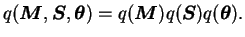(5.51)

The distributions of the state variables are as they were in the individual models,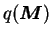as in Equation (5.14) and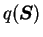as in Equations (5.43)-(5.46). The distribution of the parameters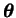is the product of corresponding distributions of the individual models, i.e.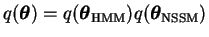.

There is one additional approximation in the choice of the form of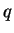. This can be clearly seen from Equation (5.50). After marginalising over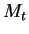, the conditional probability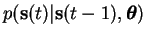will be a mixture of as many Gaussians as there are states in the HMM. Marginalising out the past will result in an exponentially growing mixture thus making the problem intractable.

Our ensemble learning approach solves this problem by using only a single Gaussian as the posterior approximation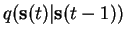. This is of course just an approximation but it allows a tractable way to solve the problem.Next: The algorithm Up: Combining the two models Previous: The structure of the   Contents
Antti Honkela 2001-05-30